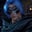Related Tags

communitycreator
javascript

# How to get the median of an array of numbers in JavaScriptGutha Vamsi Krishna

In this shot, we will learn how to get the median of an array of numbers in JavaScript.

The median is the middle value of the given list of data when it is arranged in order.

### Problem statement

Given an array of numbers, find the median.

To find the median of an array of numbers, we face two cases:

1. Odd length array
2. Even length array

### Example of an odd length array

Input: arr = [9,4,6,3,8]

Output: 6

#### Explanation

• Sort the given numbers [3,4,6,8,9].
• The middle value is 6, and that is the median.

### Example of an even length array

Input: arr = [7,4,6,9,3,8]

Output: 6

#### Explanation

• Sort the given numbers [3,4,6,7,8,9].
• Here, the middle elements are 6 and 7.
• $6 + 7$ = $13$
• $13/2 = 6.5$
• The median is 6.5.

### Solution

You can easily find the median if the number of elements is odd, but if the count is even, then we need to take an average of the two middle elements.

### Implementation

#### Case 1

Let’s first take a look at an odd length array.

//given odd number of elements
let arr = [9,4,6,3,8]

//sort the array
arr.sort((a, b) => a - b)

//declare median variable
let median;

//if else block to check for even or odd
if(arr.length%2 != 0){
//odd case

//find middle index
let middleIndex = Math.floor(arr.length/2)

//find median
median = arr[middleIndex]
}else{
//even case

//find middle index
let middleIndex = Math.floor(arr.length/2)

//find median
median = arr[middleIndex] + arr[middleIndex + 1]
}

//print median
console.log(median)

### Explanation

• Line 2: We have an array arr of numbers.

• Line 5: We use the sort() method to sort the array. The method sorts the array in alphabetical order by default, so we pass a comparator to sort according to numbers.

• Line 8: We declare a variable median that is used to store the median of the given array.

• Line 11: We check if the given array length is even or odd.

• If odd, the program executes the code block from lines 12 to 18.
• If even, the program executes the code block from lines 20 to 26.

In the case of an odd length array, find the middle index and the median, which is the element present at the index in the array.

### Case 2

Now, let’s look at an even length array.

//given even number of elements
let arr = [7,4,6,9,3,8]

//sort the array
arr.sort((a, b) => a - b)

//declare median variable
let median;

//if else block to check for even or odd
if(arr.length%2 != 0){
//odd case

//find middle index
let middleIndex = Math.floor(arr.length/2)

//find median
median = arr[middleIndex]
}else{
//even case

//find middle index
let middleIndex = Math.floor(arr.length/2)

//find median
median = (arr[middleIndex] + arr[middleIndex - 1])/2
}

//print median
console.log(median)

RELATED TAGS

communitycreator
javascript

CONTRIBUTORGutha Vamsi Krishna
RELATED COURSES

View all Courses

Keep Exploring

Learn in-demand tech skills in half the time